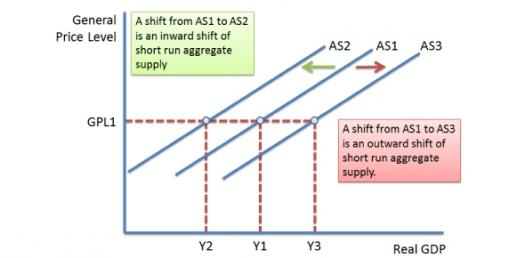# Aggregate Demand And Supply

Approved & Edited by ProProfs Editorial Team
At ProProfs Quizzes, our dedicated in-house team of experts takes pride in their work. With a sharp eye for detail, they meticulously review each quiz. This ensures that every quiz, taken by over 100 million users, meets our standards of accuracy, clarity, and engagement.
| Written by Jessicaann471
J
Jessicaann471
Community Contributor
Quizzes Created: 1 | Total Attempts: 199
Questions: 8 | Attempts: 199SettingsChap 16 & 17- Economics Course Companion (Oxford)

• 1.

### Refer to the diagram. Assume that wages are initially set on the basis of price level P1 and that the economy is operating at its full-employment level of Qf. The short-run effect of an increase in demand is best reflected by a move from:

• A.

Point e to point g

• B.

Point e to point b

• C.

Point e to point a

• D.

Point e to point c

A. Point e to point g
Explanation
In the diagram, point e represents the initial equilibrium where wages are set based on price level P1 and the economy is operating at full employment level Qf. An increase in demand will lead to an increase in output and employment in the short run. This will cause a shift in the aggregate demand curve to the right, resulting in a move from point e to point g. At point g, both output and price level will be higher than at point e, reflecting the short-run effect of an increase in demand.

Rate this question:

• 2.

### Refer to the diagram above. If P1 is the price level and Qf is the full-employment output, then the long-run aggregate supply curve:

• A.

Is AS1

• B.

Connects points a and e

• C.

Is AS2

• D.

Connects points e, b, and d

D. Connects points e, b, and d
Explanation
The long-run aggregate supply curve connects points e, b, and d because these points represent different levels of output at the full-employment level. Point e represents the full-employment output level, while points b and d represent output levels above and below full employment, respectively. The curve connects these points to show the relationship between price level and output in the long run.

Rate this question:

• 3.

### Refer to the diagram. Assume aggregate demand is initially given by AD1 and cost-push inflation pushes the aggregate supply curve from AS1 to AS2. If the government uses fiscal policy to restrain the inflation:

• A.

Aggregate demand will increase to AD2

• B.

Aggregate supply will shift even further to the left

• C.

Aggregate demand will shift to the left

• D.

The economy will move from point b to point a

C. Aggregate demand will shift to the left
Explanation
If the government uses fiscal policy to restrain inflation, it will typically involve reducing government spending and/or increasing taxes. This will lead to a decrease in aggregate demand as consumers and businesses have less money to spend. As a result, the aggregate demand curve will shift to the left, causing a decrease in overall spending in the economy.

Rate this question:

• 4.

### Refer to the diagram. If Q1 represents the full-employment output, then the curve labeled B is the:

• A.

LRAS curve

• B.

SRAS curve

B. SRAS curve
Explanation
The curve labeled B in the diagram represents the SRAS curve. SRAS stands for Short-Run Aggregate Supply, which shows the relationship between the price level and the quantity of goods and services that firms are willing and able to supply in the short run. The SRAS curve is upward sloping, indicating that as the price level increases, firms are willing to produce and supply more output. In this context, if Q1 represents the full-employment output, it means that at this level of output, the SRAS curve intersects with the aggregate demand curve, representing equilibrium in the short run.

Rate this question:

• 5.

### If the economy is initially at a point on its long-run aggregate supply curve, a decrease in aggregate demand will:

• A.

Permanently increase unemployment

• B.

Temporarily increase unemployment

• C.

Permanently increase inflation

• D.

Temporarily increase inflation

B. Temporarily increase unemployment
Explanation
A fall in aggregate demand will temporarily reduce the rate of inflation and increase unemployment.

Rate this question:

• 6.

• 7.

• 8.

### What is Aggregate Supply?

Related TopicsBack to top
×

Wait!
Here's an interesting quiz for you.